February 9, 2017

# 1 tip for effective data visualization in Python

Yes, you read correctly — this post will only give you 1 tip. I know most posts like this have 5 or more tips. I once saw a post with 15 tips, but I may have been daydreaming at the time. You're probably wondering what makes this 1 tip so special. "Vik", you may ask, "I've been reading posts that have 7 tips all day. Why should I spend the time and effort to read a whole post for only 1 tip?" I can only answer that data visualization is about quality, not quantity. Like me, you probably spent hours learning about all the various charts that are out there — pie charts, line charts, bar charts, horizontal bar charts, and millions of others. Like me, you thought you understood data visualization. But we were wrong. Because data visualization isn't about making different types of fancy charts. It's about understanding your audience and helping them achieve their goals. Oh, this is embarrassing — I just gave away the tip. Well, if you keep reading, I promise that you'll learn all about making effective data visualization, and why this one tip is useful. By the end, you'll be able to make useful plots like this: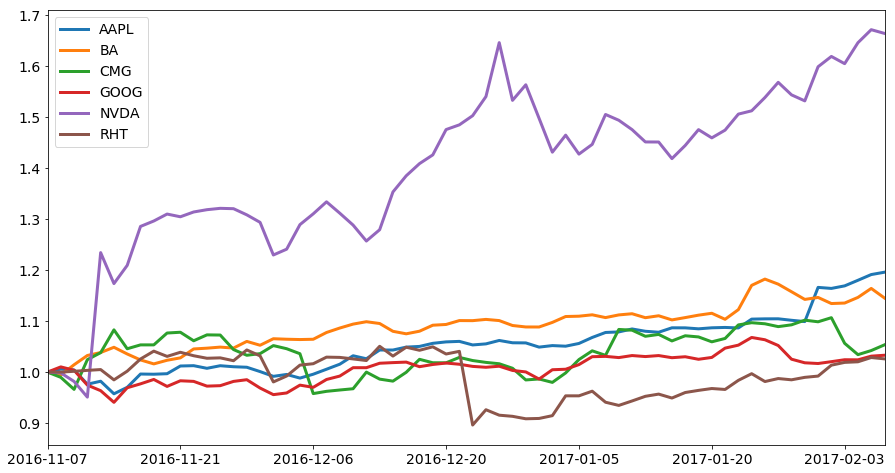Let's start by talking about some data visualizations in your daily life that may surprise you. Did you know that whenever you see a weather map on TV, check the time on your wall clock, or stop at a traffic light, you're seeing a visual representation of numeric data? Don't believe me? Let's dive a little more into how a wall clock shows time. When the time is

`5:05`, you don't see the actual time on the clock. Instead, you see a small "hand" pointing to `5`, and a big "hand" pointing to `1`, like this: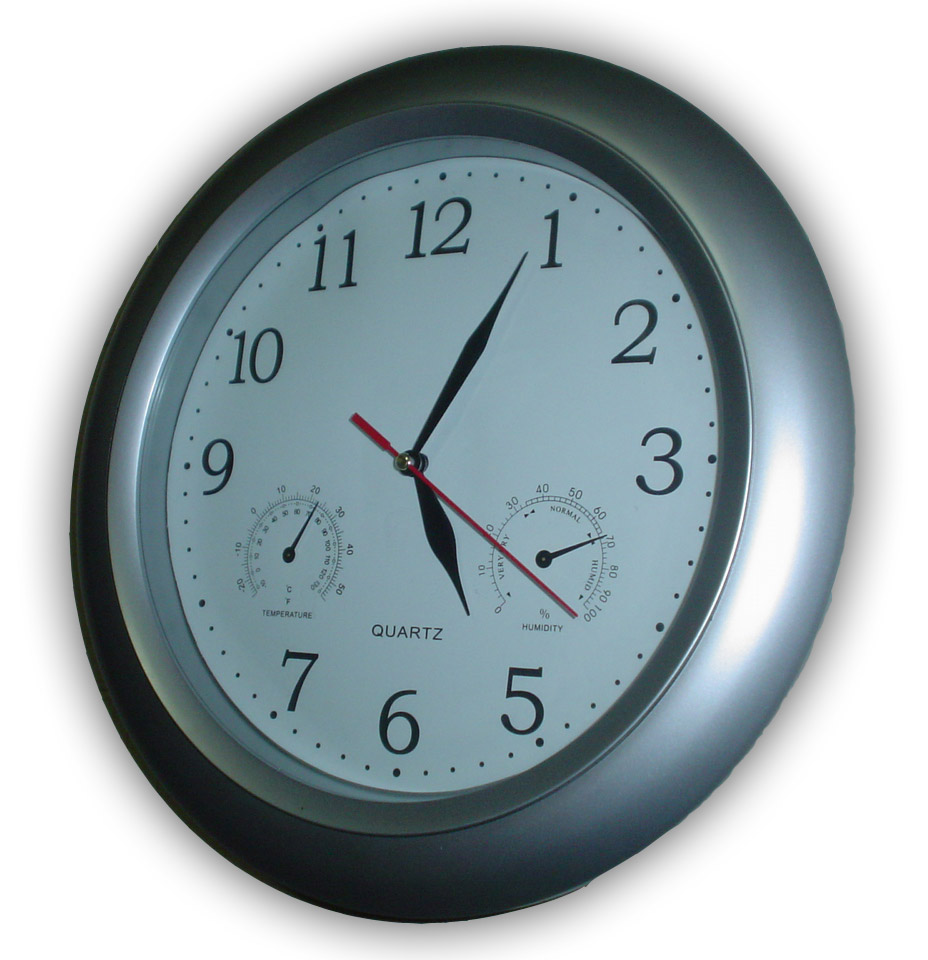We've been trained to translate from this visual representation of the data to a time,

`5:05`. Wall clocks are unfortunately an example of data visualization that makes it harder to understand the underlying data. It takes much more mental effort to parse the time on a wall clock than it does for a digital clock. Wall clocks were created before displaying the time on a digital display was possible, so the only solution was displaying the time via two "hands". Let's look at a visualization that makes it much easier to understand the underlying data, the weather map. Lets look at this map as an example: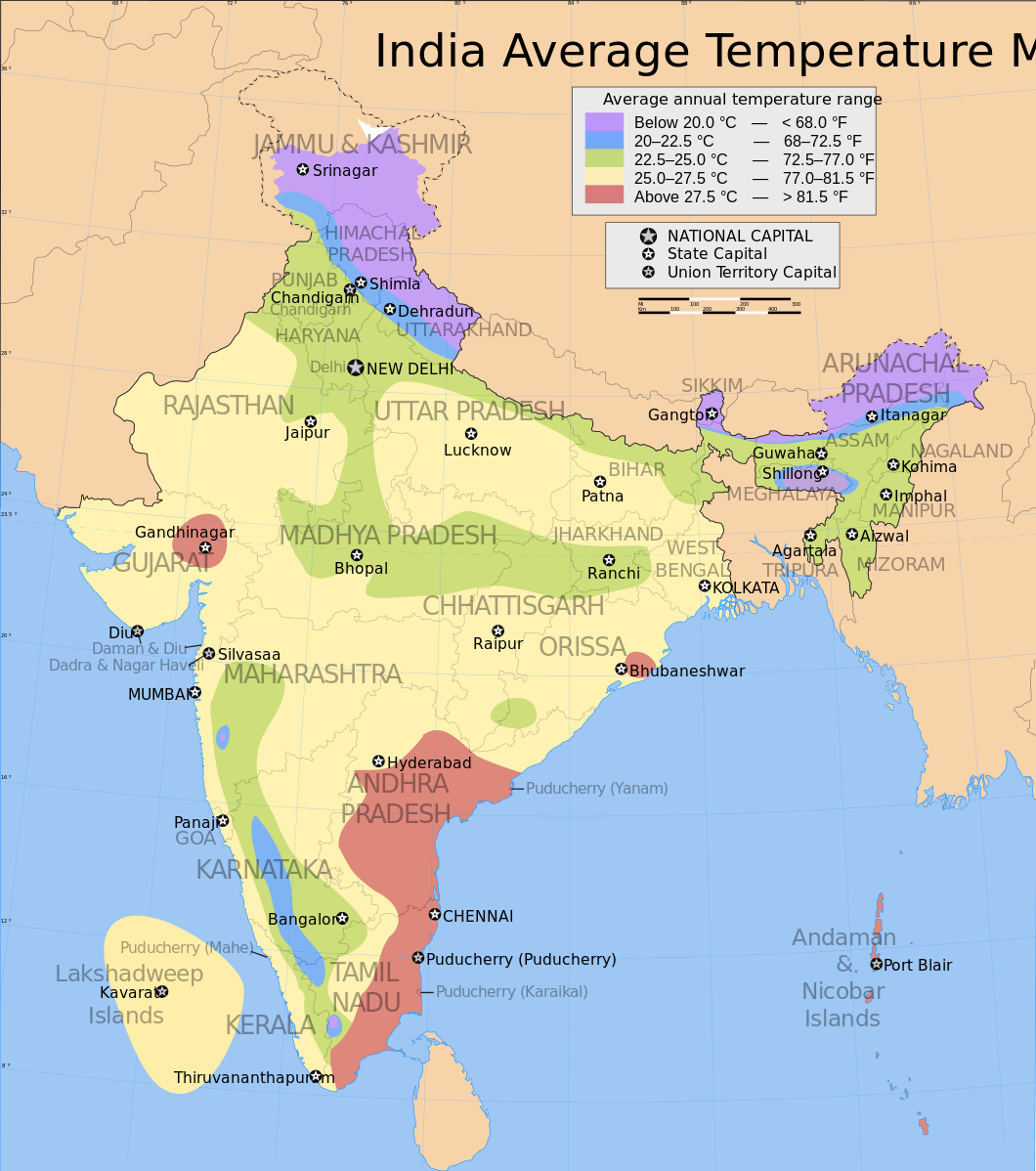Looking at the map above, you can instantly tell that the coasts of Andhra Pradesh and Tamil Nadu are some of the hottest places in India. Arunachal Pradesh and Jammu and Kashmir are some of the coldest. We can see the "lines" along which higher average temperatures transition to lower average temperatures. The map is great for looking at geographic temperature trends despite display issues with the map — some labels overflow their boxes, or are too light. If we had instead represented this as a table, we would have "lost" a significant amount of data. For example, from the map, we can quickly tell that Hyderabad is colder than the coast of Andhra Pradesh. In order to communicate all of the information in the map, we'd need a table full of temperature data for every place in India, like this but longer:

City Average Annual Temperature
1 Chennai 29.5
2 Raipur 26.0
3 New Delhi 23.0

This table is hard to think of in geographic terms. Two cities next to each other in the table might be right next to each other geographically, or extremely far apart. It's hard to figure out geographic trends when you're looking at one city at a time, so the table isn't useful for looking at high level geographic temperature changes. However, the table is extremely useful for looking up the average temperature of your city — far more useful than the map. You can instantly tell that the average annual temperature of Hyderabad is

`27.0` degrees Celsius. Understanding what representations of the data are useful in which contexts is critical for creating effective data visualizations.

### What we've learned so far

• Visualizations aren't always better than numbers for representing data.
• Even a visualization that doesn't look good can be effective if it matches the goals of the audience.
• Effective visualization can enable viewers to discover patterns that they could never find using numeric representations.

In this post, we'll learn how to make effective visualizations by walking through visualizing the performance of our investment portfolio. We'll represent the data a few different ways, and talk about the pros and cons of each approach. Too many tutorials start with making charts, but never discuss

why those charts are being made. At the end of this post, you'll have more insight into what charts are useful in which situations, and be able to more effectively communicate using data. If you want to go more in depth, you should try our courses on exploratory data visualization and storytelling through data visualization. We'll be using Python 3.5 and Jupyter notebook in case you want to follow along.

## Tabular representations of the data

Let's say that we own a few shares of stock, and we want to track their performance:

• `AAPL` — 500 shares
• `GOOG` — 450 shares
• `BA` — 250 shares
• `CMG` — 200 shares
• `NVDA` — 100 shares
• `RHT` — 500 shares

We bought all of the shares on November 7th, 2016, and we want to track their performance to date. We first need to download the daily share price data, which we can do with the

yahoo-finance package. We can install the package using `pip install yahoo-finance`. In the below code, we:

• Import the `yahoo-finance` package.
• Loop through each symbol

• Download data from `2016-11-07` to the previous day.
• Extract the closing prices for each day.
• Create a dataframe with all of the price data.
• Display the dataframe.
``````
from yahoo_finance import Share
import pandas as pd
from datetime import date, timedelta

symbols = ["AAPL", "GOOG", "BA", "CMG", "NVDA", "RHT"]

data = {}
days = []
for symbol in symbols:
share = Share(symbol)
yesterday = (date.today() - timedelta(days=1)).strftime("%Y-%m-%d")
prices = share.get_historical('2016-11-7', yesterday)
close = [float(p["Close"]) for p in prices]
days = [p["Date"] for p in prices]
data[symbol] = close

stocks = pd.DataFrame(data, index=days)
``````
AAPL BA CMG GOOG NVDA RHT
2017-02-08 132.039993 163.809998 402.940002 808.380005 118.610001 78.660004
2017-02-07 131.529999 166.500000 398.640015 806.969971 119.129997 78.860001
2017-02-06 130.289993 163.979996 395.589996 801.340027 117.309998 78.220001
2017-02-03 129.080002 162.399994 404.079987 801.489990 114.379997 78.129997
2017-02-02 128.529999 162.259995 423.299988 798.530029 115.389999 77.709999

As you can see above, this gives us a table where each column is a stock symbol, each row is a date, and each cell is the price of that stock symbol on that date. The entire dataframe has

`62` rows. This is very good if we want to lookup the price of a specific stock on a specific day. For example, I can quickly tell that `AAPL` shares cost `128.75` at market close on February 1st, 2017. However, we might only care about if we've made or lost money off of each stock symbol. We can find the difference between the price of each share when we bought it, and the current price. In the below code, we subtract the stock prices when we bought them from the current stock prices.

``````
change = stocks.loc["2017-02-06"] - stocks.loc["2016-11-07"]
change
``````
``````
AAPL    19.879989
BA      20.949997
CMG     13.100006
GOOG    18.820007
NVDA    46.040001
RHT      1.550003
dtype: float64
``````

Great! It looks like we made money on every investment. However, we can't tell by what percentage our investments have increased. We can do this with a slightly more complex formula:

``````
pct_change = (stocks.loc["2017-02-06"] - stocks.loc["2016-11-07"]) / stocks.loc["2016-11-07"]
pct_change
``````
``````
AAPL    0.180056
BA      0.146473
CMG     0.034249
GOOG    0.024051
NVDA    0.645994
RHT     0.020217
dtype: float64
``````

It looks like our investments have done extremely well percentage-wise. But it's hard to tell how much money we've made overall. Let's multiply the price change by our share counts to see how much we've made:

``````
import numpy as np
share_counts = np.array([500, 250, 200, 450, 100, 500])
portfolio_change = change * share_counts
portfolio_change
``````
``````
AAPL    9939.99450
BA      5237.49925
CMG     2620.00120
GOOG    8469.00315
NVDA    4604.00010
RHT      775.00150
dtype: float64
``````

``sum(portfolio_change)``
``31645.49969999996``

And look at our purchase price to eyeball how much we've made on a percentage basis:

``sum(stocks.loc["2016-11-07"] * share_counts)``
``565056.50745000003``

We've gotten pretty far with numeric data representations. We were able to figure out how much our portfolio value increased. In many cases, data visualization isn't necessary, and a few numbers can express everything you want to share. In this section, we learned that:

• Numeric representations of data can be enough to tell a story.
• It's good to try to simplify tabular data when you can before moving to visualization.
• Understanding the goals of your audience is important to effectively representing data.

Numeric representations stop working well when you want to find patterns or trends in your data. Let's say we wanted to figure out if any stocks were more volatile in December, or if any stocks went down then back up. We could try to use measures like

standard deviation, but they wouldn't give us the whole story:

``stocks.std()``
``````
AAPL     6.135476
BA       6.228163
CMG     15.352962
GOOG    21.431396
NVDA    11.686528
RHT      3.225995
dtype: float64
``````

The above tells us that

`68%` of `AAPL` closing share prices in our time period are within `5.54` of the mean price. It's hard to tell if this indicates low or high volatility, though. It's also hard to tell if `AAPL` has recently increased in price or not. In the next section, we'll figure out how to visualize our data to identify these hard to quantify trends.

## Plotting all our stock symbols

The first thing we can do is make a plot of each stock series. We can do this by using the

pandas.DataFrame.plot method. This will create a line plot of the daily closing prices for each stock symbol. We need to first sort the dataframe in reverse order, as currently, it is sorted in descending order of date, and we want it to be in ascending order:

``````

stocks = stocks.iloc[::-1]
stocks.plot()
``````
``<matplotlib.axes._subplots.AxesSubplot at 0x1105cd048>``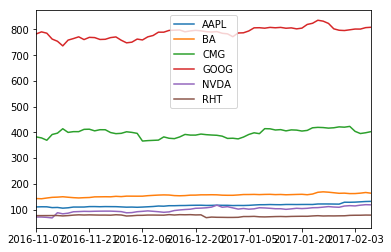The above plot is a good start, and we've come very far in a short amount of time. Unfortunately, the chart is a bit cluttered, and it's hard to tell the overall trends for some of the lower priced symbols. Let's normalize the chart to show each daily closing price as a fraction of the starting price:

``````
normalized_stocks = stocks / stocks.loc["2016-11-07"]
normalized_stocks.plot()
``````
``<matplotlib.axes._subplots.AxesSubplot at 0x10f81b8d0>``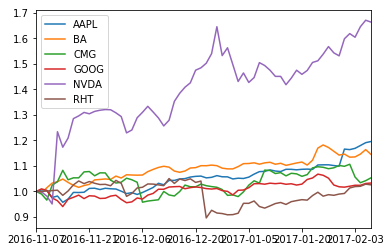This plot is much better for seeing relative trends in each stock price. Each line shows us how the value of the stock is changing relative to its purchase price. This shows us which of our stocks are increasing on a percentage basis, and which ones aren't. We can see that the price of

`NVDA` shares increased very steeply soon after we bought it, and have continued to increase in value. `RHT` seems to have lost quite a bit of value at the end of December, but the price has been recovering steadily. Unfortunately, there are some visual issues with this plot that make the plot hard to read. The labels are squished together, and it's hard to see what happens to `GOOG`, `CMG`, `RHT`, `BA`, and `AAPL` since the lines are bunched together. We'll increase the size of the plot using the `figsize` keyword argument, and increase the width of the lines to fix these issues. We'll also increase the axis label and axis font sizes to make them easier to read.

``````
import matplotlib.pyplot as plt

normalized_stocks.plot(figsize=(15,8), linewidth=3, fontsize=14)
plt.legend(fontsize=14)``````
``<matplotlib.legend.Legend at 0x10eaba160>``In the above plot, it's far easier to separate the lines visually since we have more space, and they're thicker. The labels are also easier to read since they're larger. Let's say that we want to see how much of our total portfolio value is in each stock over time, on a percentage basis. We'd need to first multiply each stock price series by the number of shares we hold, then divide by the total portfolio value, then make an area plot. This would let us see if some stocks are increasing enough to constitute a much larger share of our overall portfolio. In the below code, we:

• Multiply each stock price by the number of shares we hold to get the total worth of the shares we own of each symbol.
• Divide each row by the total value of the portfolio on that date to figure out what percentage of our portfolio value each stock is.
• Plot the values in an area plot, where the y axis goes from `0` to `1`.
• Hide the y axis labels.
``````
portfolio = stocks * share_counts
portfolio_percentages = portfolio.apply(lambda x: x/sum(x), axis=1)
portfolio_percentages.plot(kind="area", ylim=(0,1), figsize=(15,8), fontsize=14)
plt.yticks([])
plt.legend(fontsize=14)``````
``<matplotlib.legend.Legend at 0x10ea8cda0>``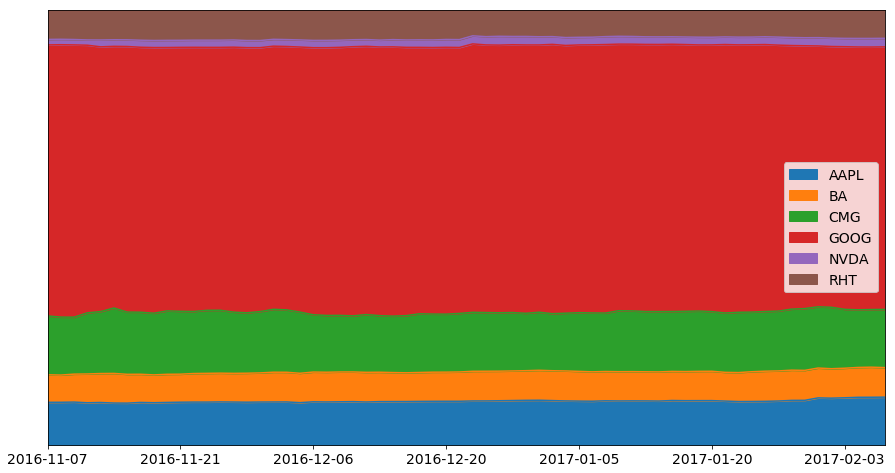As you can see above, most of our portfolio's value is in

`GOOG` stock. The overall allocation of dollars per stock symbol hasn't changed much since we purchased them. From looking at the data in a different way earlier, we know that the price of `NVDA` has grown quite quickly in the past few months, but from this view, we can see that its total value isn't that much of our portfolio. This means that although the stock price of `NVDA` has grown substantially, it hasn't had a huge affect on our overall portfolio value. Note how the chart above is fairly hard to parse and see trends in. This is an example of a chart that's usually better as a series of numbers that show the average percentage of portfolio value each stock comprises. A good way to think about this is "what questions can we answer better with this chart than with any other chart?" If the answer is "no questions", then you're probably better off with something else. To get a better handle on our overall portfolio value over time, we can plot it out:

``portfolio.sum(axis=1).plot(figsize=(15,8), fontsize=14, linewidth=3)``
``<matplotlib.axes._subplots.AxesSubplot at 0x110dede48>``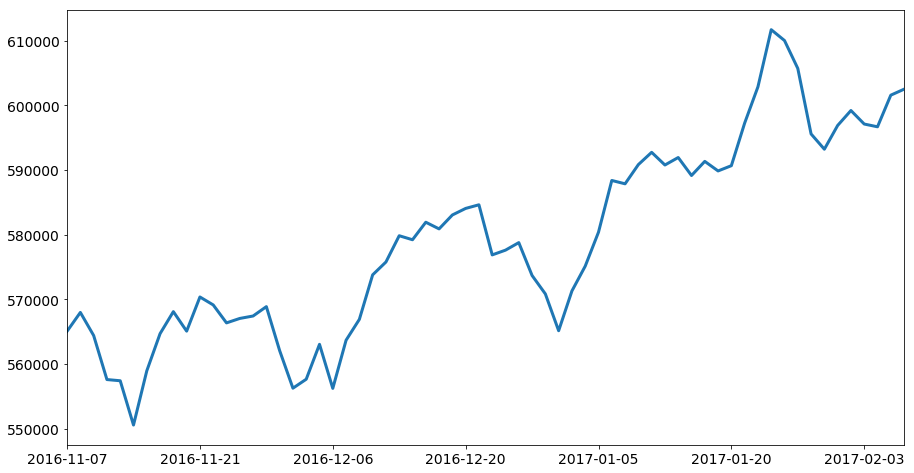When looking at the above plot, we can see that our portfolio lost a good amount of money around the beginning of November, the end of December, and the end of January. When we look at some of our previous plots, we can discover that this is mostly due to drops in the prices of

`GOOG`, which is most of our portfolio value. Being able to visualize the data from different angles helps us untangle the story of our overall portfolio, and answer questions more intelligently. For instance, making these plots helped us figure out:

• The overall portfolio value trends.
• Which stocks make up what percentage of our portfolio value.
• The movement of the individual stocks.

Without understanding all three points, we wouldn't be able to figure out why the price of our portfolio is changing. To tie this all the way back to the tip we started this post with, understanding your audience and the questions they'll ask will help you design visualizations that meet their goals. The key to effective visualization is ensuring that it helps your audience understand complex tabular data more easily.

## Next steps

In this post, you've learned:

• How to simplify numeric data.
• What questions can be answered with numeric data, and what questions require visualizations.
• How to frame visualizations to answer questions.
• Why we make visualizations in the first place.
• Why some charts aren't as useful to our audience.

If you want to go into more depth, and learn more about how to explore data and tell stories using data, you should check out our two courses on